﻿ 质量分布对弹簧振子链系统振动传递特性的影响
 舰船科学技术2023, Vol. 45Issue (1): 31-36    DOI: 10.3404/j.issn.1672-7649.2023.01.006PDF

Effect of mass distribution on vibration transmission characteristics of spring oscillator chain system
ZHAO Peng, JI Gang, LI Zong-wei
College of Naval Architecture and Ocean Engineering, Naval University of Engineering, Wuhan 430033, China
Abstract: In order to study the general regularity of mass distribution on vibration transmission characteristics of one-dimensional spring oscillator chain system, the mechanism about passband and stopband of periodic spring oscillator chain system is analyzed from the perspective of wave transmission, and the mathematical theory of random matrix product is used to reveal transmission characteristics of disordered mass distribution system. The finite element method is used to calculate frequency response of spring oscillator chain model with the same mass and mass disorder, and the variation of subunit vibration amplitude at each frequency is obtained to compare with theoretical analysis value. The study shows that disordered distribution of masses has a great improvement on vibration transmission performance and the system will no longer have a passband. Vibration will be mainly confined near the excitation source and the localization factor theoretical formula can respond well to the vibration decay. The results help to understand vibration localization phenomenon of non-periodic systems and provide a reference for the vibration localization analysis of multidimensional complex structures.
Key words: spring oscillator chain     mass disorder     vibration transfer     localization factor
0 引　言

1 一维单耦合结构的波传递特性分析

 ${y_j} = \left[ {\begin{array}{*{20}{c}} \lambda &{} \\ {}&{{\lambda ^{ - 1}}} \end{array}} \right]{y_{j - 1}} = W{y_{j - 1}}。$ (1)

 ${y_N} = {W^N}{y_0} ，$ (2)

 ${W^N} = \left[ {\begin{array}{*{20}{c}} {{e^{ + iNk}}}&{} \\ {}&{{e^{ - iNk}}} \end{array}} \right] ，$ (3)

 ${W^N} = \left[ {\begin{array}{*{20}{c}} {{e^{ + N\alpha }}}&{} \\ {}&{{e^{ - N\alpha }}} \end{array}} \right] 。$ (4)

 $\prod\limits_{j = 1}^n {{W_j} = {W_1} \cdots {W_N}} 。$ (5)

 ${y_{j + 1}} = \left[ {{W_j}} \right]{y_j} = \left[ {\begin{array}{*{20}{c}} {\dfrac{1}{{{t_j}}}}&{ - \dfrac{{{r_j}}}{{{t_j}}}} \\ { - \dfrac{{r_j^*}}{{t_j^*}}}&{\dfrac{1}{{t_j^*}}} \end{array}} \right]{y_j}。$ (6)

 $\prod\limits_{j = 1}^n {{W_j}} = \left[ {\begin{array}{*{20}{c}} {\dfrac{1}{{{t_N}}}}&{ - \dfrac{{{r_N}}}{{{t_N}}}} \\ { - \dfrac{{r_N^*}}{{t_N^*}}}&{\dfrac{1}{{t_N^*}}} \end{array}} \right]。$ (7)

 $\gamma = \mathop {\lim }\limits_{N \to \infty } - \dfrac{1}{N}\ln \left| {{t_N}} \right| = \dfrac{1}{2}{\sigma ^2}\dfrac{{{\partial ^2}\left( {\ln \left| {\dfrac{1}{{t(\alpha )}}} \right|} \right)}}{{{\partial ^2}\alpha }} + o(\sigma _\alpha ^2) 。$ (8)

2 弹簧振子链系统的波动分析及局域化因子计算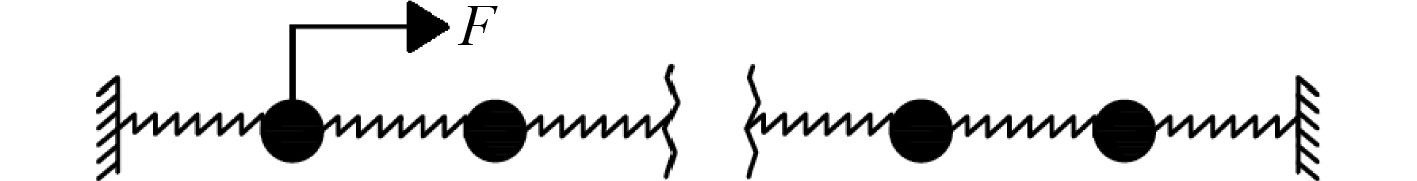图 1 弹簧振子链系统 Fig. 1 Mass-spring system

 $\left( {\begin{array}{*{20}{c}} {{x_{j + 1}}} \\ {{x_j}} \end{array}} \right) = \left[ {\begin{array}{*{20}{c}} {2 - \dfrac{{{\omega ^2}m}}{k}}&{ - 1} \\ 1&0 \end{array}} \right]\left( {\begin{array}{*{20}{c}} {{x_j}} \\ {{x_{j - 1}}} \end{array}} \right) ，$ (9)

 ${\boldsymbol{W}} = \left[ {\begin{array}{*{20}{c}} \lambda &{} \\ {}&{{\lambda ^{ - 1}}} \end{array}} \right] ，$ (10)
 $\lambda = \frac{{2 - {\omega ^2}m/k + \sqrt {{{\left( {2 - {\omega ^2}m/k} \right)}^2} - 4} }}{2} 。$ (11)

$0 < \omega < 2k/m$ 时， $\lambda$ 为复数，表征波动可以无损传播，对应周期系统的通频带。

 ${\gamma _m} = {\left. {\frac{1}{4}{\sigma ^2}\frac{{{\partial ^2}\ln \left( {1 + {\delta ^2}} \right)}}{{{\partial ^2}\mu }}} \right|_{\mu = 1}} = \frac{{m{\omega ^2}{\sigma ^2}}}{{8k{{\sin }^2}k}}。$ (12)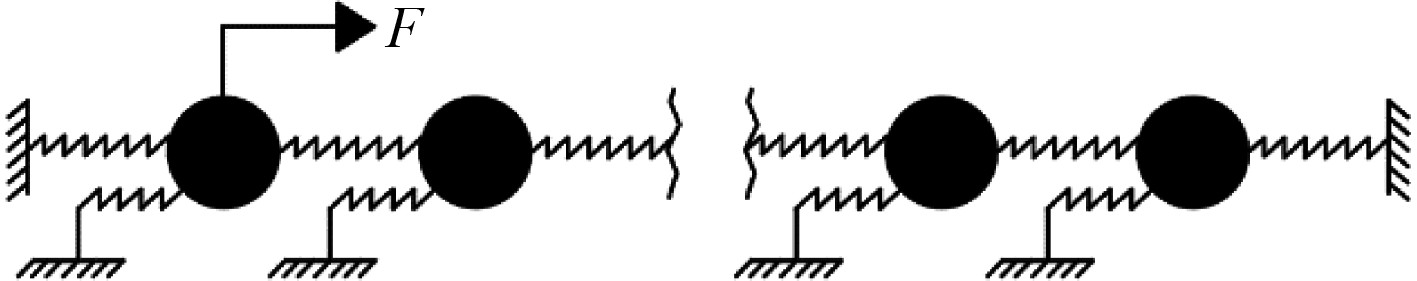图 2 含有对地刚度的弹簧振子链系统 Fig. 2 Mass-spring system with ground stiffness

 $\left( {\begin{array}{*{20}{c}} {{x_j}} \\ {{x_{j + 1}}} \end{array}} \right) = \left[ {\begin{array}{*{20}{c}} 0&1 \\ { - 1}&{\dfrac{{ - m{\omega ^2} + {k_d}}}{k} + 2} \end{array}} \right]\left( {\begin{array}{*{20}{c}} {{x_{j - 1}}} \\ {{x_j}} \end{array}} \right)，$ (13)

 ${\boldsymbol{T}} = {\boldsymbol{q}}\left[ {\begin{array}{*{20}{c}} \lambda &{} \\ {}&{{\lambda ^{ - 1}}} \end{array}} \right]{{\boldsymbol{q}}^{ - 1}}，$ (14)

${{\boldsymbol{y}}_j} = {{\boldsymbol{q}}^{ - 1}}{{\boldsymbol{x}}_j} = {\left[ {\begin{array}{*{20}{c}} {{L_j}}&{{R_j}} \end{array}} \right]^{\rm{T}}}$ ，则单元之间的波动关系可以表示为：

 ${{\boldsymbol{y}}_j} = {\boldsymbol{W}}{{\boldsymbol{y}}_{j - 1}} = \left[ {\begin{array}{*{20}{c}} \lambda &{} \\ {}&{{\lambda ^{ - 1}}} \end{array}} \right]{{\boldsymbol{y}}_{j - 1}} 。$ (15)

 $\left\{ {\begin{array}{*{20}{c}} {\lambda = p + 1 - \sqrt {p\left( {p + 2} \right)} }，\\ {{\lambda ^{ - 1}} = p + 1 + \sqrt {p\left( {p + 2} \right)}。} \end{array}} \right.$ (16)

 $\cos k = 1 - \frac{{{\omega ^2} - \omega _0^2}}{{2V}}。$ (17)

 ${\gamma _m} = {\left. {\frac{1}{4}{\sigma ^2}\frac{{{\partial ^2}\ln \left( {1 + {\delta ^2}} \right)}}{{{\partial ^2}\mu }}} \right|_{\mu = 1}} = \frac{1}{2}\frac{{{\sigma ^2}{\omega ^4}}}{{4{V^2} - {{({\omega ^2} - \omega _0^2 - 2V)}^2}}} ，$ (18)

 ${\gamma _m} = \frac{1}{2}\frac{{{\sigma ^2}{\omega ^4}}}{{4{V^2} - {{({\omega ^2} - 2V)}^2}}}。$ (19)
3 弹簧振子链波动传递特性理论验证

3.1 简单周期弹簧振子链系统的波动传递特性验证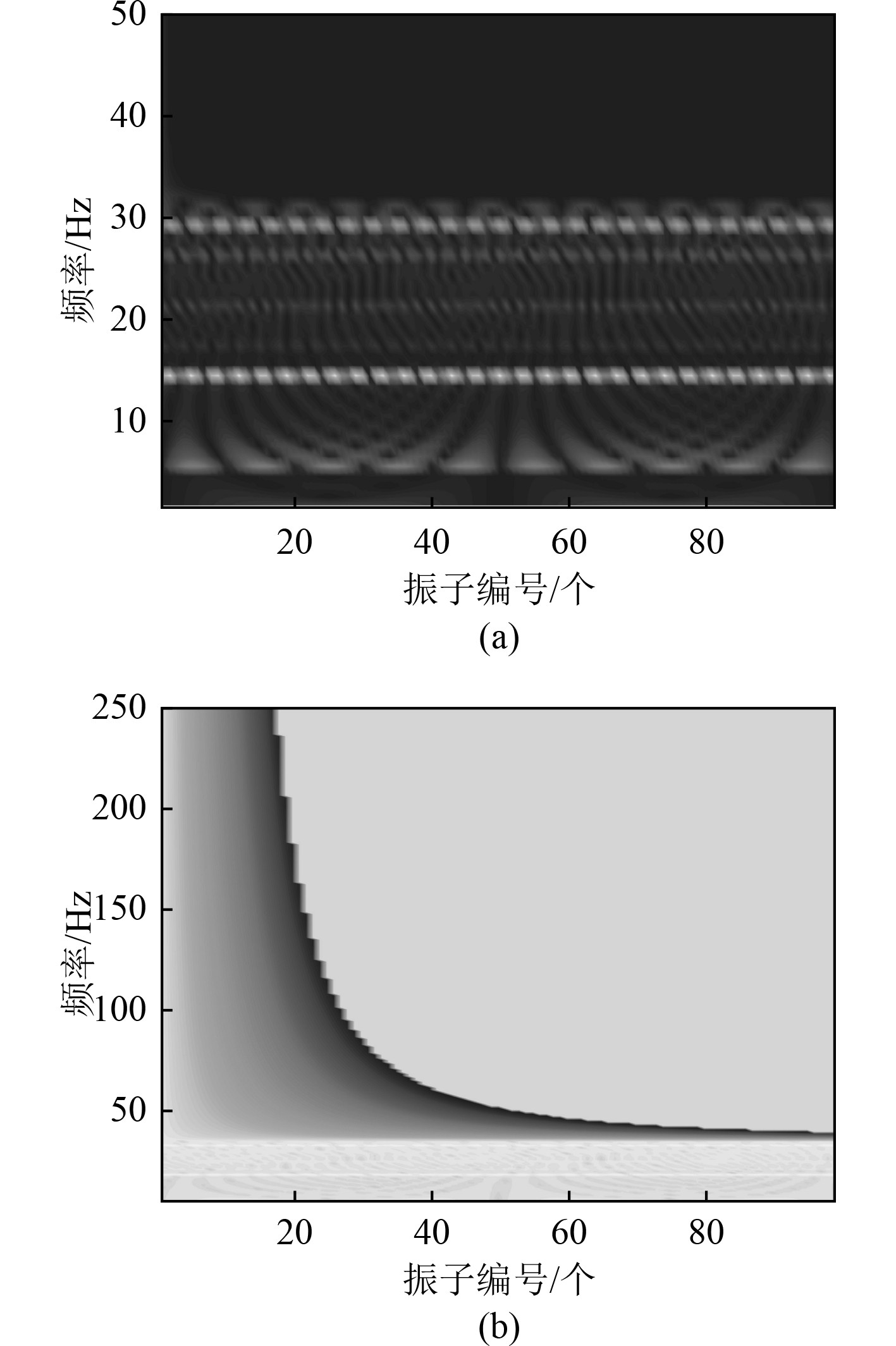图 3 两种形式的能量色谱图 Fig. 3 Two different kinds of contour maps

3.2 简单弹簧振子链质量无序分布的局域化效应验证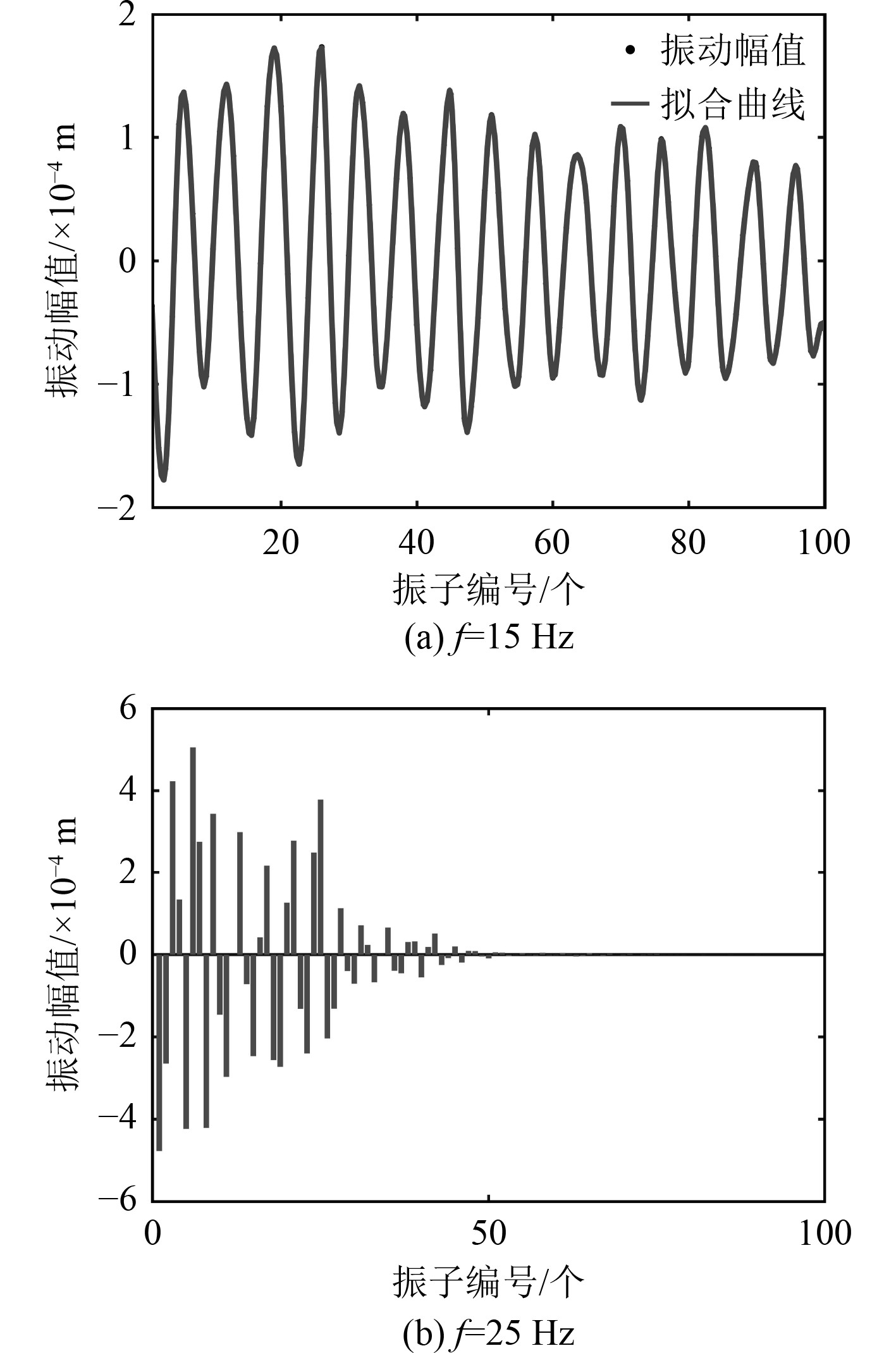图 4 质量无序分布的振动幅值图 Fig. 4 Vibration amplitudes with disorder mass distribution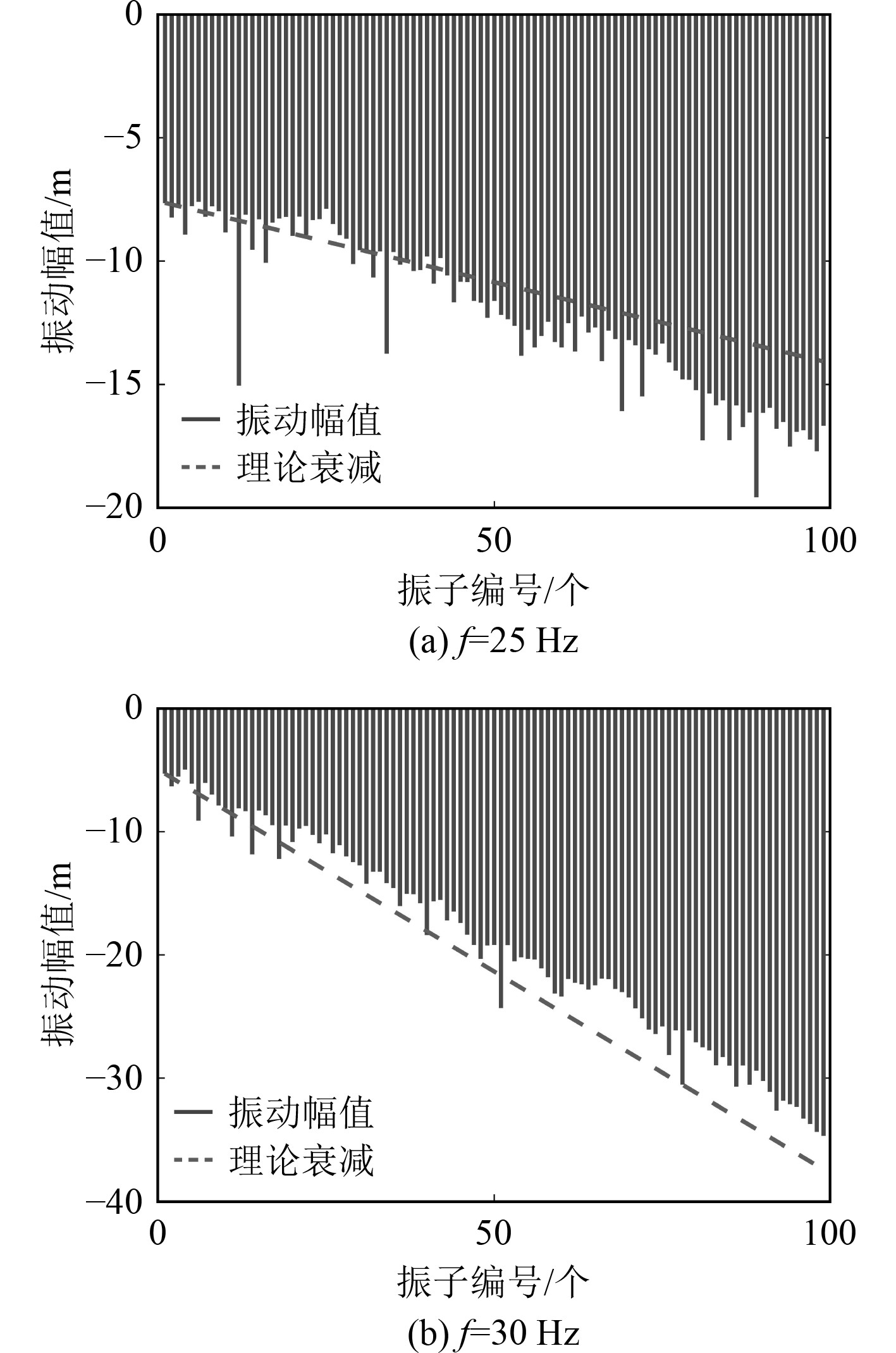图 5 振动幅值曲线同理论局域化因子的比较 Fig. 5 Comparisons of vibration amplitudes and theoretical results
3.3 含有对地刚度的弹簧振子链质量无序分布的局域化效应验证表 1 弹簧振子链模型相关参数 Tab.1 Related parameters of spring oscillator chains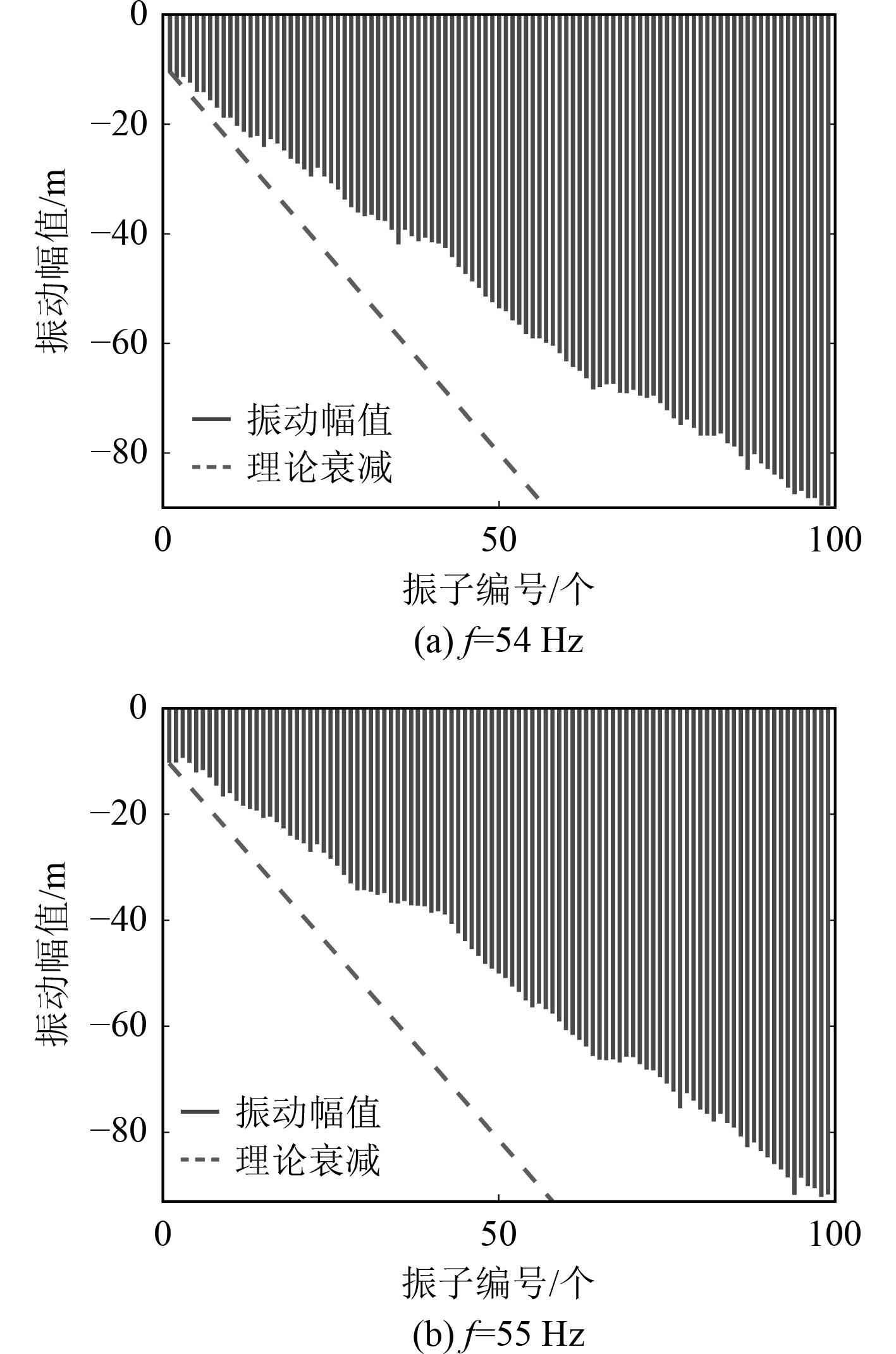图 6 振动幅值曲线同理论局域化因子的比较 Fig. 6 Comparisons of vibration amplitudes and theoretical results

4 结　语

1）对于具有相同质量分布的周期弹簧振子链系统，在频域上存在通频带，振动可以无衰减传播。但当对振子质量进行无序配置后，结构变成非周期系统，在频域上全部表现为止频带特征。

2）基于波传递理论和Furstenberg定理以及系统的状态传递矩阵可以推导出局域化因子的近似解，理论公式反映了大量子单元条件下衰减效应的平均结果。有限元实例模型的仿真情况充分验证了理论公式的可靠性。

3）理论预报公式在实际工程中具有指导意义，通过增加系统的无序程度 ${\sigma ^2}$ ，可增强结构的局域化效应，使振动局限在小片区域，提升结构声学性能。

  王毅泽. 周期结构中弹性波的色散关系与振动局部化问题研究[D]. 哈尔滨. 哈尔滨工业大学, 2006.  纪刚, 李宗威, 周其斗. 不等间距加筋圆柱壳的振动局域化研究[J]. 振动工程学报, 2021, 34(3): 592-600. JI Gang, LI Zong-wei, ZHOU Qi-dou. Localization of vibration on a disordered framed cylindrical shell[J]. Journal of Vibration Engineering, 2021, 34(3): 592-600. DOI:10.16385/j.cnki.issn.1004-4523.2021.03.017  谭路, 纪刚等. 结构不等间距布置对圆柱壳结构声学性能的影响[J]. 振动与冲击, 2017, 36(24): 189-194. TAN Lu, JI Gang, et al. Influence of unequal spacing arrangement of structures on structural acoustic characteristics of cylindrical shells[J]. Journal of Vibration and Shock, 2017, 36(24): 189-194. DOI:10.13465/j.cnki.jvs.2017.24.029  ANDERSON P W. Absence of diffusion in certain random lattices[J]. Physical Review, 1958, 109(5): 1492-1505. DOI:10.1103/PhysRev.109.1492  HODGES, C. H, WOODHOUSE. Confinement of vibration by one-dimensional disorder, I: theory of ensemble averaging[J]. Journal of Sound and Vibration, 1989, 130(2): 237-251. DOI:10.1016/0022-460X(89)90552-X  HODGES C. H, WOODHOUSE J. Confinement of vibration by one-dimensional disorder II: a numerical experiment on different ensemble averages[J]. Journal of Sound and Vibration, 1989, 130(2): 253-268. DOI:10.1016/0022-460X(89)90553-1  FAULKNER M G, HONG D P. Free vibrations of a mono-coupled periodic system[J]. Journal of Sound and Vibration, 1985, 99(1): 29-42. DOI:10.1016/0022-460X(85)90443-2  FURSTENBERG H. Noncommuting Random Products[J]. Transactions of the American Mathematical Society, 1963, 108(3): 377-428. DOI:10.1090/S0002-9947-1963-0163345-0  KISSEL G J. Localization in disordered periodic structures[D]. Ph D Dissertation, Massachusetts Institute of Technology, 1988.  BOUZIT D, PIERRE C. Vibration confinement phenomena in dis-ordered, mono-coupled, multi-span beams[J]. Journal of Vibration and Acoustics, 1992, 114(4): 521-530. DOI:10.1115/1.2930294  李宗威, 纪刚, 周其斗. 非周期系统的局域化现象研究[J]. 中国舰船研究, 2020, 15(3): 88-94. LI Zong-wei, JI Gang, ZHOU Qi-dou. Localization phenomena in disordered periodic systems[J]. Chinese Journal of Ship Research, 2020, 15(3): 88-94. DOI:10.19693/j.issn.1673-3185.01591  BALUNI V, WILLEMSEN J. Transmission of acoustic waves in a random layered medium[J]. Physical Review A, 1985, 31(5): 3358-3363.  李宗威, 纪刚, 周其斗. 多自由度系统基于单通带近似法的振动局域化分析[J]. 中国舰船研究, 2020, 15(6): 143-148. LI Zong-wei, JI Gang, ZHOU Qi-dou. Analysis on vibration localization of a multi-DOF system based on the single band approximation method[J]. Journal of Ship Research, 2020, 15(6): 143-148. DOI:10.19693/j.issn.1673-3185.01811